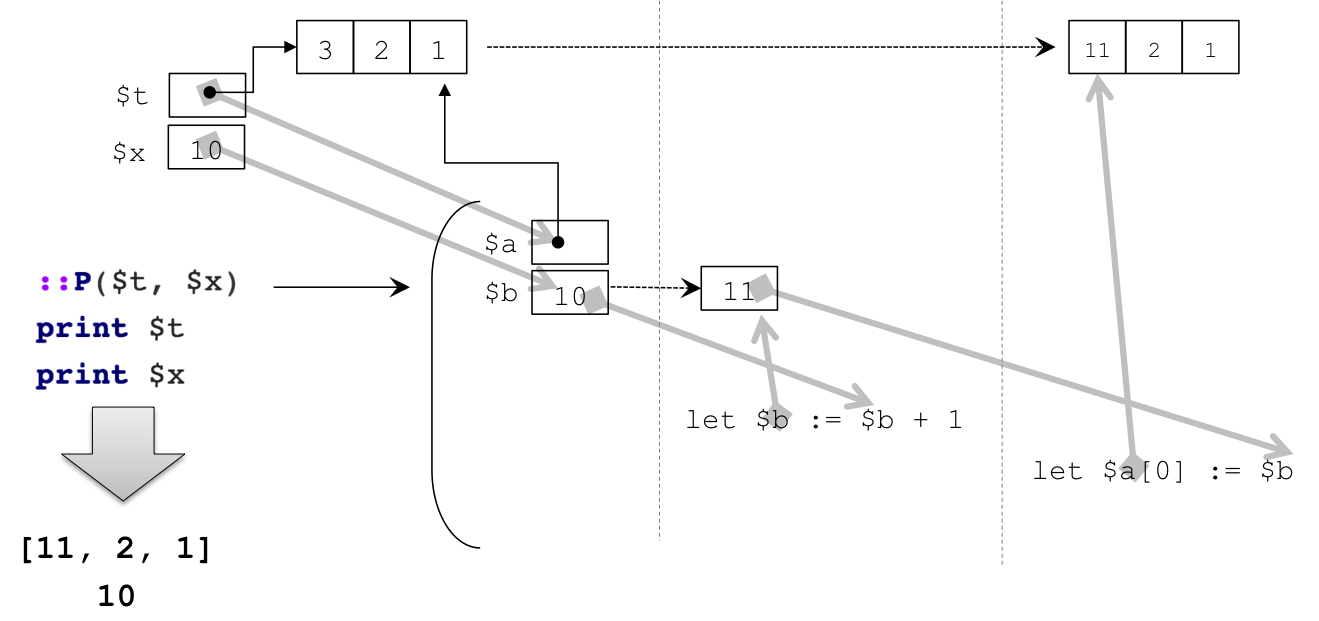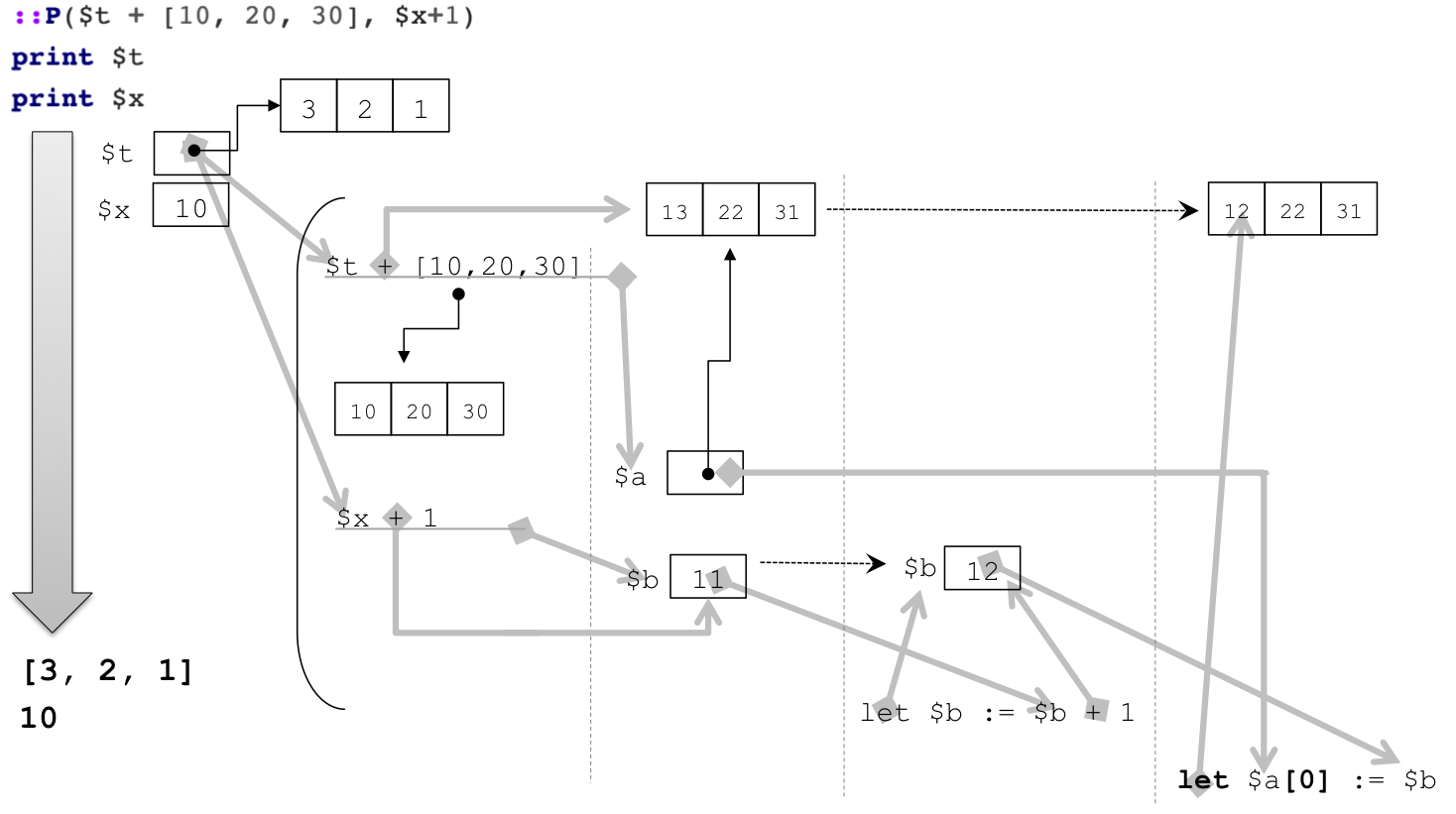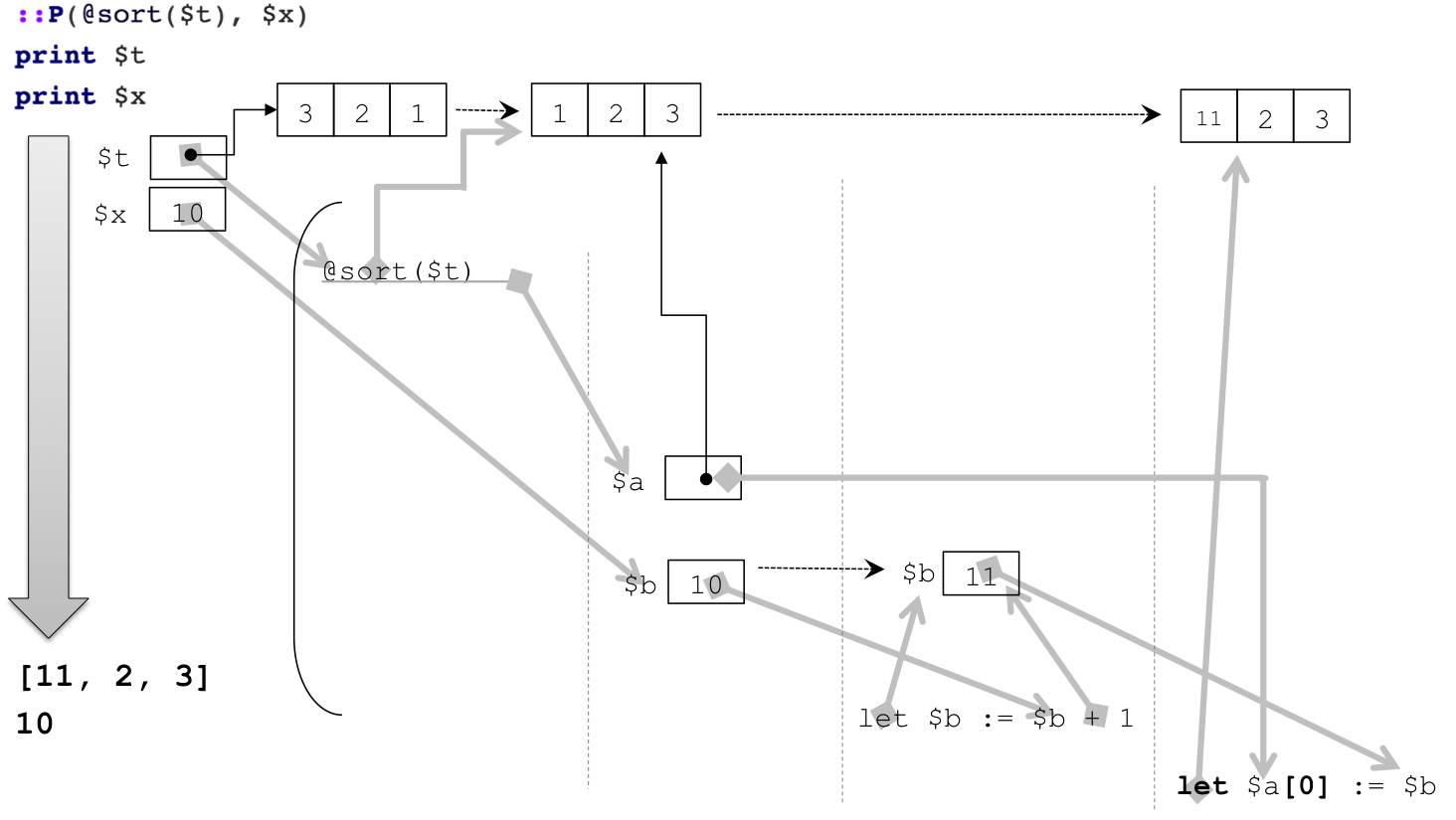## Argument evaluation strategies¶

Programming languages through the years have introduced several strategies to determine when and how to evaluate the argument of a routine call (by a routine, we mean a sequence of code that can be called with parameters like functions, procedures, methods, processes, coroutines, etc.). When a routine is called, its code is run with the parameters substituted by the actual arguments provided during the call. What differs is the exact nature of the substitution.

Call-by-value replaces the parameters in the routine body with the value of the arguments. In other words, the expressions in arguments are evaluated and this value is bound to the routine's parameters seen as local variables of the routine. Thus, the callee cannot modify a value referred to by the caller through its argument. This strategy is the most common strategy, used in C, Scheme, etc.

Call-by-reference replaces the parameters by a reference to the value of the arguments. The reference is handled transparently in the routine body, where parameters are used like local variables. This strategy allows a routine to alter a value refered by the caller. This strategy is available in Pascal, Fortran, C++ (using a reference for the type of the parameter), etc.

Call-by-name replaces the parameters in the routine body with the expressions given as actual arguments. This strategy is not very common in programming languages (Algol60 introduced it), but corresponds to the macro mechanism, with the exception of the time of evaluation (calling-by-name is interleaved with evaluation, while macros are textual substitutions done before any evaluation, which may lead to differences when a routine definition can be the product of the evaluation).

This categorization is blurred by the nature of the programming languages: declarative (e.g. purely functional) or imperative, the type system (if any; consider the C++ approach where arguments are passed by values, but a reference type exists), the representation of values (if all values are ‟boxed〞and implemented as pointers to boxes, the call by value cannot be distinguished from the call by reference), and additional mechanisms (like lazy evaluation, memoization or futures and promises), etc.

### Antescofo Evaluation Strategy¶

Antescofo implements call-by-value for functions, process and objects; and Antescofo macros implement textual substitution, which loosely corresponds to call-by-name.

However, nota bene that Antescofo data structures, i.e. tab, map, nim and string, are implemented through a reference to an underlying memory area. So, for instance, a tab may be shared between the caller and the callee even if the call is by value. This ends up with a behavior like call-by-reference for data structures. This behavior "call-by-value where the value is a reference" is common: this is for instance the behavior in C, C++ or Java.

The following example illustrates this point. Consider the following process and variable definitions:

@proc_def ::P($a,$b)
{
let $b :=$b + 1
let $a :=$b
}

$t := [3, 1, 2]$x := 10


the corresponding data layout:Then, the call

::P($t,$x)
print $t print$x


will produce:

  [11, 2, 1]
10


The evaluation is sketched in the following diagramThe value of $t is copied into the parameter $a but this value is a reference to an underlyng memory zone where the tab is actually stored. So, when the first element of the tab is updated in the process, this is visible in the value referenced by $t. On the other hand, $x still refers to the value 10 because this value is fully copied into parameter $b and a subsequent change does not affect the value refered by $x.

Consider now the call:

::P($t + [10, 20, 30],$x+1)
print $t print$x


it will produce:

  [3, 2, 1]
10


The values referred to by $t and $x are untouched by the evaluation of ::P which is sketched in the following diagram:This time, the values in the caller are unaffected by the execution of ::P. As a matter of fact, expression $t +[10, 20, 30] creates a new tab and it it this new tab that is mutated by the element assignment in the process. The consequence is that the value refered by $t remains unaltered.

As a final example, examine the following expression:

::P(@sort($t),$x)
print $t print$x


it will produce:

  [11, 2, 3]
10


This time, the argument @sort($t) modifies the tab referred by $t in place and returns its argument. So the tab processed by ::P is also the tab referred by \$t.Notice that the previous examples involve processes but the same behavior is achieved with functions. Nota bene that scalars are never modified through a function or a process call.